Daily Practice Problems
Class 6 Maths
AlgebraQuestion 1:

Give expression for the following:

(a) 12 subtracted from z.

(c) p multiplied by 16.

(d) y divided by 8

Question 2:

If the side of an equilateral triangle is 3x, find its perimeter.

Question 3:

Complete the table and by inspection of the table, find the solution to the equation x -11 = 6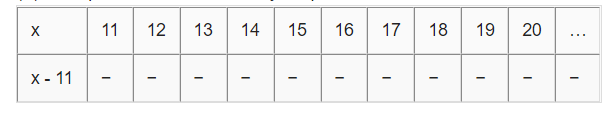Question 4:

Fill in the blanks:

(a) 5 added to -5 = ______

(b) If x = 3, then 3x – 5 = _____

(c) If x = 1 and y = 2, then 2x + 3y = _____

(d) If 10x – 6 = 14, then x = _____

(e) 4 less than a number x = _____

Question 5:

A starts his car from Delhi at 6.00 am to Amritsar. The uniform speed of his car is x km/h. At 12.00 noon, he finds that he is still 50 km away from Amritsar. Find the distance between Delhi and Amritsar.

Question 6:

Anshika’s Score in Science is 25 more than the two-third of her score in Sanskrit. If she scores x marks in Sanskrit, find her score in Science.

Question 7:

Here is a pattern of houses with matchsticks: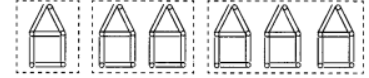Question 8:

Think of a number, add 2 to it and then multiply the sum by 6, the result is 42.

Question 9:

The side of a regular hexagon is s cm. Find its perimeter.

Question 10:

State which of the following are equations with a variable. In the case of equations with a variable, identify the variable.

(a) x + 20 = 70

(b) 8 × 3 = 24

(c) 2p > 30

(d) n – 4 = 100

(e) 20b = 80

(f)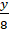< 50

Question 11:

If x = 3, find the value of the following:

(i) x – 7

(ii))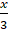– 1

Question 12:

Get the algebraic expressions in the following cases using variables, constants and arithmetic operations.

(i) Number 5 added to three times the product of number m and n.

(ii) Product of numbers y and z subtracted from 10.

(iii)Sum of numbers x and y subtracted from their product.

Question 13:

Complete the table and find the solution of the equation 19 – x = 13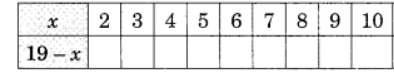Question 14:

True and False statement:

(i)  x=-3 is the solution of the equation x + 4=1

(ii) 0 is the solution of the equation x + 1 = 1

(iii) x=20 is the solution of the equation 2x + 5 = 25

(iv) The three sides of the triangle are a,a,b. Then perimeter of the triangle is (a+b).

Question 15:

If a = 3, b =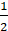and c =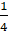, find the value of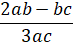Question 16:

Give expressions in the following cases:

(a) 5 is added to 4 times a number x

(b) Twice a number y subtracted from 9

(c) 6 times y to which 3 is added

(d) 5 is added to the sum of x and y

(e) n multiplied by 5 and 2 subtracted from the product

Question 17:

Think of a number x. Multiply it by 3 and add 5 to the product and subtract y subsequently. Find the resulting number.

Question 18:

State which of the following are equations with a variable?

(a) 12 = x – 5

(b) 2x > 7

(c)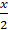= 5

(d) 5 + 7 = 3 + 9

(e) 7 = (11 x 5) – (12 x 4)

Question 19:

The teacher distributes 5 pencils per student. Can you tell how many pencils are needed, given the number of students? (Use ‘s’ for number of students.)

Questions 20:

Write which letters give us the same rule as that given by L.

**********